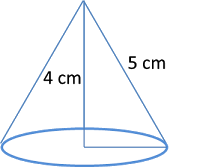# GRE® Solid Geometry Practice

Concept: Sector of circle rotated or recast into a cone

This GRE quant practice question is a geometry - solid geometry problem solving question. Computing the volume of a right circular cone obtained by recasting or rotating a sector of a circle.

#### Question: A sector of a circle of radius 5 cm is recast into a right circular cone of height 4 cm. What is the volume of the resulting cone?

1. 12 π cm3
2. 100 π cm3
3. 33 π cm3
4. 32 π cm3
5. 4 π cm3

Video Explanation will be added soon

### 3 steps to the answer

1. The radius of the sector of the circle will be the slant height of the cone
2. Compute the base radius of the cone
3. Find the volume of the cone

### Step 1: Find slant height of the cone

The diagram below shows the sector of a circle with radius 5 cm.When recast into a right-circular cone of height 4 cm the sector will look as shown in the diagram given below.The radius of the sector will be the slant height of the cone.
So, the slant height of the cone is 5 cm.

### Step 2: Compute the base radius of the coneAs can be seen in the diagram, the base radius, slant height, and the height of the cone form a right triangle, with the slant height being the hypotensue of the triangle.
We know the slant height of the cone, which is the hypotenuse of the triangle is 5 cm.
The height of the cone, which is one of the perpendicular sides of the triangle is 4 cm.

Using Pythagoras theorm, we can compute the radius r = $$sqrt{$\text{slant height}$^2 - $text{height}^2}$
r = $\sqrt{5^2-4^2}$ = 3 cm

### Step 3: Find the volume of the cone

The volume of a right circular cone is $\frac{1}{3}$πr2h
r = 3; h = 4
So, volume = $\frac{1}{3}$π x 32 x h = 12 π cm3